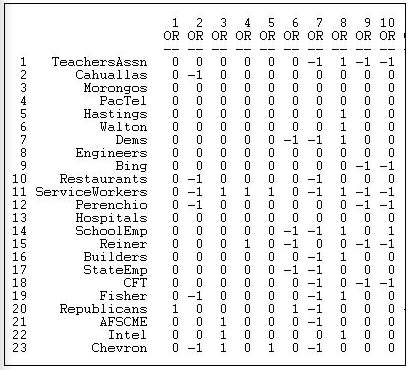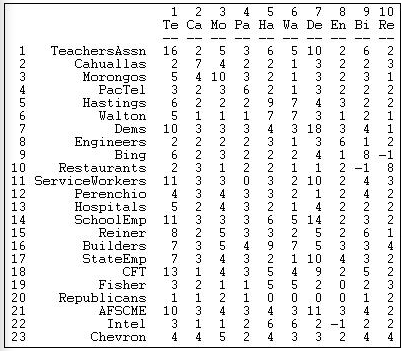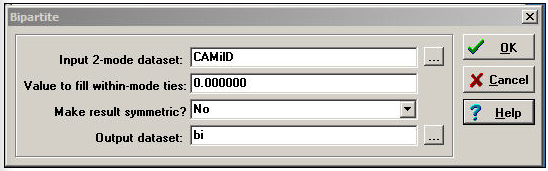$$\newcommand{\id}{\mathrm{id}}$$ $$\newcommand{\Span}{\mathrm{span}}$$ $$\newcommand{\kernel}{\mathrm{null}\,}$$ $$\newcommand{\range}{\mathrm{range}\,}$$ $$\newcommand{\RealPart}{\mathrm{Re}}$$ $$\newcommand{\ImaginaryPart}{\mathrm{Im}}$$ $$\newcommand{\Argument}{\mathrm{Arg}}$$ $$\newcommand{\norm}{\| #1 \|}$$ $$\newcommand{\inner}{\langle #1, #2 \rangle}$$ $$\newcommand{\Span}{\mathrm{span}}$$

# 17.2: Bipartite Data Structures

$$\newcommand{\vecs}{\overset { \rightharpoonup} {\mathbf{#1}} }$$ $$\newcommand{\vecd}{\overset{-\!-\!\rightharpoonup}{\vphantom{a}\smash {#1}}}$$$$\newcommand{\id}{\mathrm{id}}$$ $$\newcommand{\Span}{\mathrm{span}}$$ $$\newcommand{\kernel}{\mathrm{null}\,}$$ $$\newcommand{\range}{\mathrm{range}\,}$$ $$\newcommand{\RealPart}{\mathrm{Re}}$$ $$\newcommand{\ImaginaryPart}{\mathrm{Im}}$$ $$\newcommand{\Argument}{\mathrm{Arg}}$$ $$\newcommand{\norm}{\| #1 \|}$$ $$\newcommand{\inner}{\langle #1, #2 \rangle}$$ $$\newcommand{\Span}{\mathrm{span}}$$ $$\newcommand{\id}{\mathrm{id}}$$ $$\newcommand{\Span}{\mathrm{span}}$$ $$\newcommand{\kernel}{\mathrm{null}\,}$$ $$\newcommand{\range}{\mathrm{range}\,}$$ $$\newcommand{\RealPart}{\mathrm{Re}}$$ $$\newcommand{\ImaginaryPart}{\mathrm{Im}}$$ $$\newcommand{\Argument}{\mathrm{Arg}}$$ $$\newcommand{\norm}{\| #1 \|}$$ $$\newcommand{\inner}{\langle #1, #2 \rangle}$$ $$\newcommand{\Span}{\mathrm{span}}$$

The most common way of storing 2-mode data is a rectangular data matrix of actors (rows) by events (columns). Figure 17.1 shoes a portion of the valued dataset we will use here (Data>Display).Figure 17.1: Rectangular data array of California political donations data

The California Teachers Association, for example, gave donations in opposition to the 7th, 9th, and 10th ballot initiatives, and a donation supporting the 8th.

A very common and very useful approach to two-mode data is to convert it into two one-mode datasets, and examine relations within each mode separately. For example, we could create a dataset of actor-by-actor ties, measuring the strength of the tie between each pair of actors by the number of times that they contributed on the same side of initiatives, summed across the 40-some initiatives. We could also create a one-mode dataset of initiative-by-initiative ties, coding the strength of the relation as the number of donors that each pair of initiatives had in common. The Data>Affiliations tool can be used to create one-mode datasets from a two-mode rectangular data array. Figure 17.2 displays a typical dialog box.Figure 17.2: Dialog of Data>Affiliations to create actor-by-actor relations of California donors

There are several choices here.

We have selected the row mode (actors) for this example. To create an initiative-by-initiative one-mode dataset, we would have selected column.

There are two alternative methods:

The cross-product method takes each entry of the row for actor A, and multiplies it times the same entry for actor B, and then sums the result. Usually, this method is used for binary data because the result is a count of co-occurrence. With binary data, each product is 1 only if both actors were "present" at the event, and the sum across events yields the number of events in common - a valued measure of strength.

Our example is a little more complicated because we've applied the cross-product method to valued data. Here, if neither actor donated to an initiative (0 * 0 = 0), or if one donated and the other did not (0 * -1 or 0 * +1 = 0), there is no tie. If both donated in the same direction (-1 * -1 or +1 * +1 = 1), there is a positive tie. If both donated, but in opposite directions (+1 * -1 = -1), there is a negative tie. The sum of the cross-products is a valued count of the preponderance of positive or negative ties.

The minimums method examines the entries for the two actors at each event, and selects the minimum value. For binary data, the result is the same as the cross-product method (if both, or either actor is zero, the minimum is zero; only if both are one is the minimum one). For valued data, the minimums method is essentially saying: the tie between the two actors is equal to the weaker of the ties of the two actors to the event. This approach is commonly used when the original data are measured as valued.

Figure 17.3 shows the result of applying the cross-products method to our valued data.Figure 17.3: Actor-by-actor tie strengths (Figure 17.2)

The teachers association participated in 16 campaigns (the cross-product of the row with itself counts the number of events). The association took the same position on issues as the Democratic party (actor 7) ten more times than taking opposite (or no) position. The restaurant association (node 10) took an opposite position to Mr. Bing (node 9) more frequently than supporting (or no) position. Using this algorithm, we've captured much, but not all of the information in the original data. A score of -1, for example, could be the result of two actors taking opposite positions on a single issue; or, it could mean that the two actors both took positions on several issues - and, in sum, they disagreed one more time than they agreed.

The resulting one-mode matrices of actors-by-actors and events-by-events are now valued matrices indicating the strength of the tie based on co-occurrence. Any of the methods for one-mode analysis can now be applied to these matrices to study either micro structure or macro structure.

Two-mode data are sometimes stored in a second way, called the "bipartite" matrix. A bipartite matrix is formed by adding the rows as additional columns, and columns as additional rows. For example, a bipartite matrix of our donors data would have 68 rows (the 23 actors followed by the 45 initiatives) and 68 columns (the 23 actors followed by the 45 initiatives). The two actor-by-event blocks of the matrix are identical to the original matrix; the two new blocks (actors by actors and events by events) are usually coded as zeros. The Transform>Bipartite tool converts two-mode rectangular matrices to two-mode bipartite matrices. Figure 17.4 shows a typical dialog.Figure 17.4: Dialog of Transform>Bipartite for California political donations data

The value to fill within-mode ties is usually zero, so that actors are connected only by co-presence at events, and events are connected only by having actors in common.

Once data have been put in the form of a square bipartite matrix, many of the algorithms discussed elsewhere in this text for one-mode data can be applied. Considerable caution is needed in interpretation, because the network that is being analyzed is a very unusual one in which the relations are ties between nodes at different levels of analysis. In a sense, actors and events are being treated as social objects at a single level of analysis, and properties like centrality and connection can be explored. This type of analysis is relatively rare, but does have some interesting creative possibilities.

More commonly, we seek to keep the actors and events "separate" but "connected" and to seek patterns in how actors tie events together, and how events tie actors together. We will examine a few techniques for this task later in this chapter. A good first step in any network analysis though is to visualize the data.